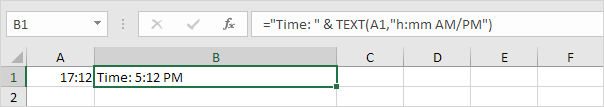# TEXT

When joining text and a number, use the TEXT function in Excel to format that number. This page contains many easy to follow TEXT function examples.

1a. For example, without using the TEXT function this would be the result.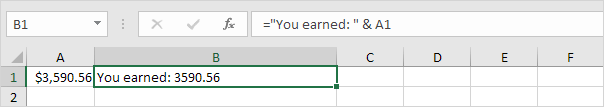1b. With the TEXT function.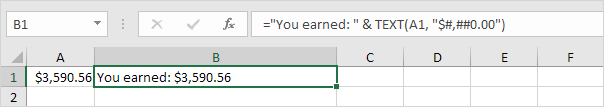Note: #,## is used to add comma's to large numbers. Always enclose the format code (second argument) in double quotation marks.

2. Here's another example. Apply a percentage format.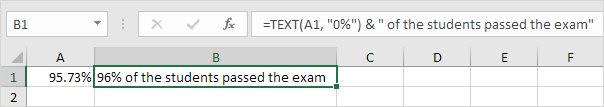Note: use 0 to display the nearest integer value. Use 0.0 for one decimal place. Use 0.00 for two decimal places, etc.

3. And another example. Format a date.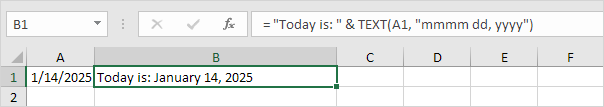Note: use less/more m's, d's and y's to change the appearance of the date.

4. For example, use the TEXT function to only display the day of the week. This time we simply use the TEXT function without adding text.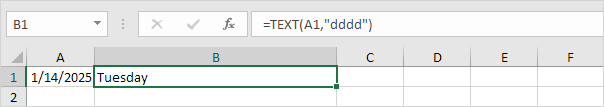5. Use the TEXT function to display a number in scientific notation.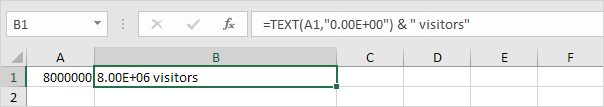6. Use the TEXT function to display a fraction and add the TRIM function to remove the leading space.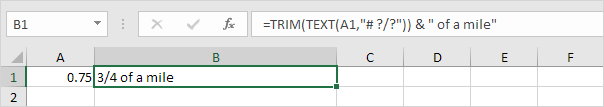Simply use the 'Format Cells' dialog box to find the right format code.

7a. For example, enter a time into cell A1 and select cell A1.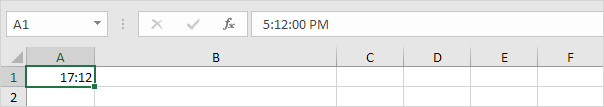7b. Right click, and then click Format Cells (or press CTRL + 1).

7c. Select the Custom category and click a format code. Next, click in the Type box, select the format code and press CTRL + c to copy this code.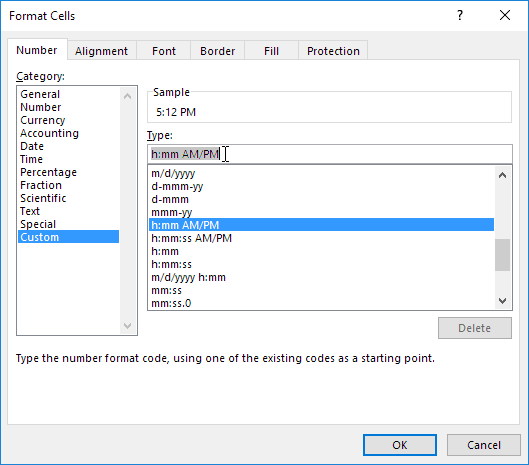Note: Excel gives you a live preview of how the time will be formatted (under Sample).

7d. Click Cancel.

7e. Paste (CTRL + v) the format code into your TEXT function. Don't forget to enclose the format code in double quotation marks.